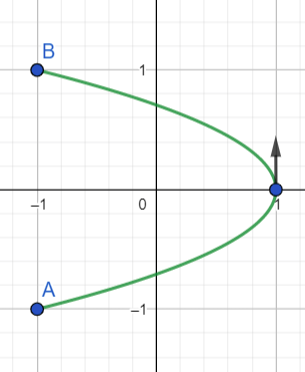# Sketch the curve by eliminating the parameter, and indicate the direction of increasing t at t=0....

## Question:

Sketch the curve by eliminating the parameter, and indicate and indicate the direction of increasing {eq}t {/eq} at {eq}t=0 {/eq}.

{eq}x=\cos (2t), y=\sin t(-\frac {\pi}{2}\leq t \leq \frac {\pi}{2}) {/eq}.

## Parametric Equations:

There are a number of ways to eliminate the parameter given a set of parametric equations, but rarely do we ever need to solve for the parameter explicitly. In the case above, we will use the trigonometric identities below to eliminate the parameter.

{eq}\begin{align*} \cos^2 t + \sin^2 t &= 1 \\ \cos 2t &= \cos^2 t - \sin^2 t \end{align*} {/eq}

We use the identities above to write

{eq}\begin{align*} x &= \cos 2t \\ &= \cos^2 t - \sin^2 t \\ &= (1 - \sin^2 t) - \sin^2 t \\ &= 1 - 2 \sin^2 t \end{align*} {/eq}

And since {eq}y = \sin t {/eq}, we have

{eq}\begin{align*} x &= 1 - 2y^2 \end{align*} {/eq}

which is an open left parabola with vertex at (1,0). And since {eq}t \in [ - \frac\pi2 , \frac\pi2 ] {/eq}, we know {eq}y \in [ \sin ( - \frac\pi2), \sin \frac\pi2 ] = [ -1,1] {/eq}. And so the start point is {eq}A= (-1,-1) {/eq} and the endpoint is {eq}B= (1, -1) {/eq}. Note that at {eq}t = 0 {/eq}, we are at the point {eq}(1,0) {/eq}, which is the vertex. At this moment the direction is straight up.